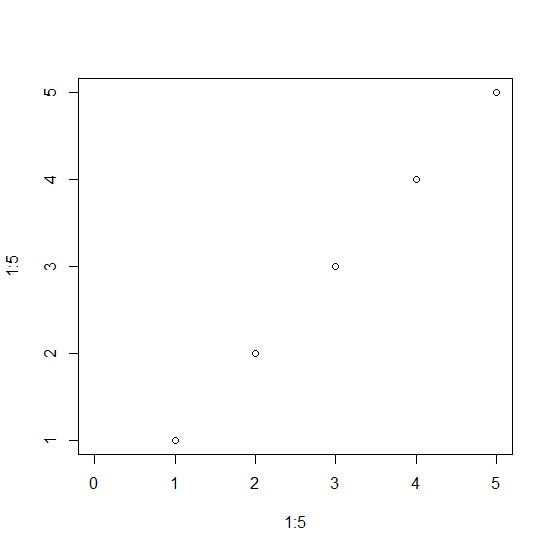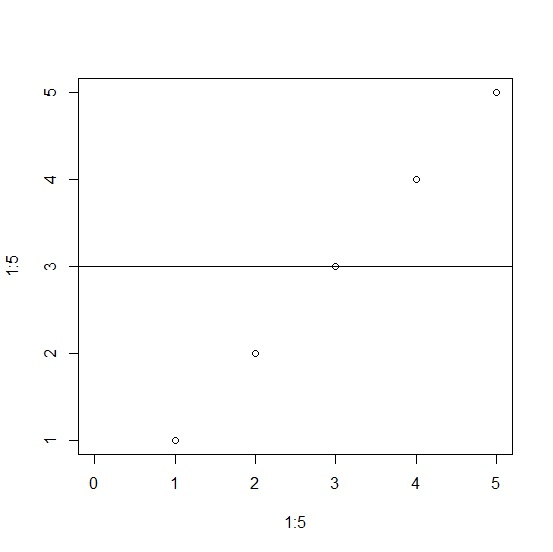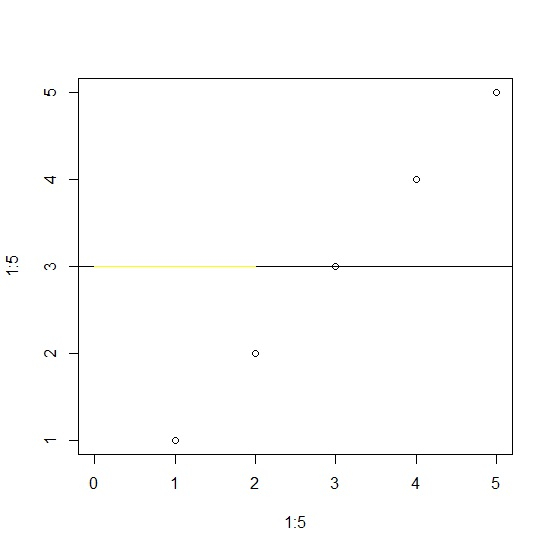# How to create horizontal lines with two different colors after a threshold in R?

To create two horizontal lines with different color after a threshold in R, we can follow the below steps −

• First of all, create a plot in base R.
• Then, add a line to the plot using abline function.
• After that, use segments function to partially change the color of the line.

## Create the plot

Using plot function to create a plot in base R −

Live Demo

plot(1:5,1:5,xlim=c(0,5))

### Output## Add a line to the plot

Using abline function to add a line to the plot −

Live Demo

plot(1:5,1:5,xlim=c(0,5))
abline(h=3)

### Output## Partially color the line

Using segments function to color the with yellow color starting from 0 to 3 −

Live Demo

plot(1:5,1:5,xlim=c(0,5))
abline(h=3)
segments(x0=0,y0=3,x1=2,y1=3,col="yellow")

#### Output